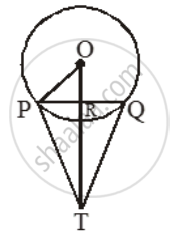Share

# In the given figure, PQ is a chord of length 8cm of a circle of radius 5cm. The tangents at P and Q intersect at a point T. Find the length TP - CBSE Class 10 - Mathematics

ConceptCircles Examples and Solutions

#### Question

In the given figure, PQ is a chord of length 8cm of a circle of radius 5cm. The tangents at P and Q intersect at a point T. Find the length TP#### Solution

Join OP and OT Let OT intersect PQ at a point R.

Then, TP = TQ and ∠PTR = ∠QTR.

∴ TR ⊥ PQ and TR bisects PQ.

∴ PR = RQ = 4 cm.

\text{Also, OR}=\sqrt{OP^{2}-PR^{2}}=\sqrt{5^{2}-4^{2})\text{ cm}

=\sqrt{25-16}=\sqrt{9}=3\text{ cm}

Let TP = x cm and TR = y cm.

From right ∆TRP, we get

TP^2 = TR^2 + PR^2

⇒ x^2 = y^2 + 16 ⇒ x^2 – y^2 = 16 …. (i)

From right ∆OPT, we get

TP^2 + OP^2 = OT^2

⇒ x^2 + 52 = (y + 3)^2 [∵ OT^2 = (OR + RT)^2 ]

⇒ x^2 – y^2 = 6y – 16 ….(ii)

From (i) and (ii), we get

6y – 16 = 16 ⇒ 6y = 32 ⇒ y = 16/3.

Putting y = 16/3 in (i), we get

\x^{2}=16+( \frac{16}{3})^{2}=(\frac{256}{9}+16)=\frac{400}{9}

\Rightarrow x=\sqrt{\frac{400}{9}}=\frac{20}{3}

Hence, length TP = x cm = 20/3 cm

= 6.67 cm.

Is there an error in this question or solution?

#### Video TutorialsVIEW ALL 

Solution In the given figure, PQ is a chord of length 8cm of a circle of radius 5cm. The tangents at P and Q intersect at a point T. Find the length TP Concept: Circles Examples and Solutions.
S